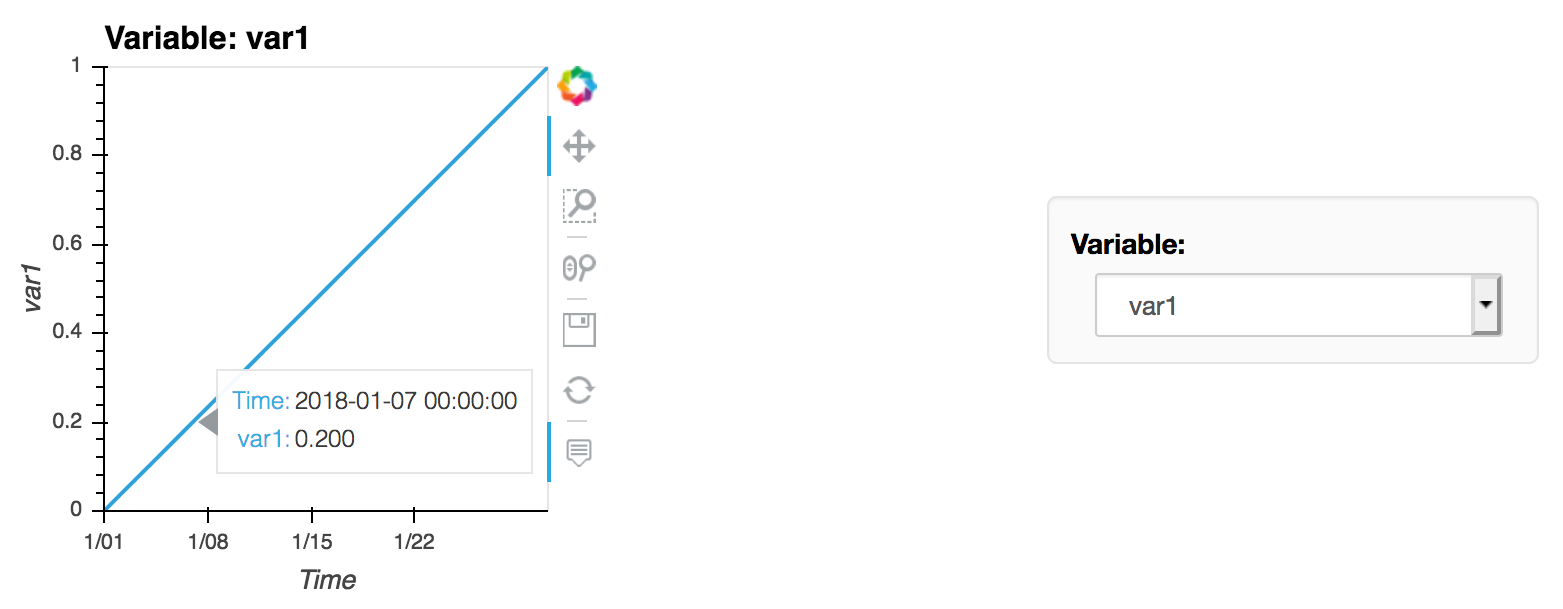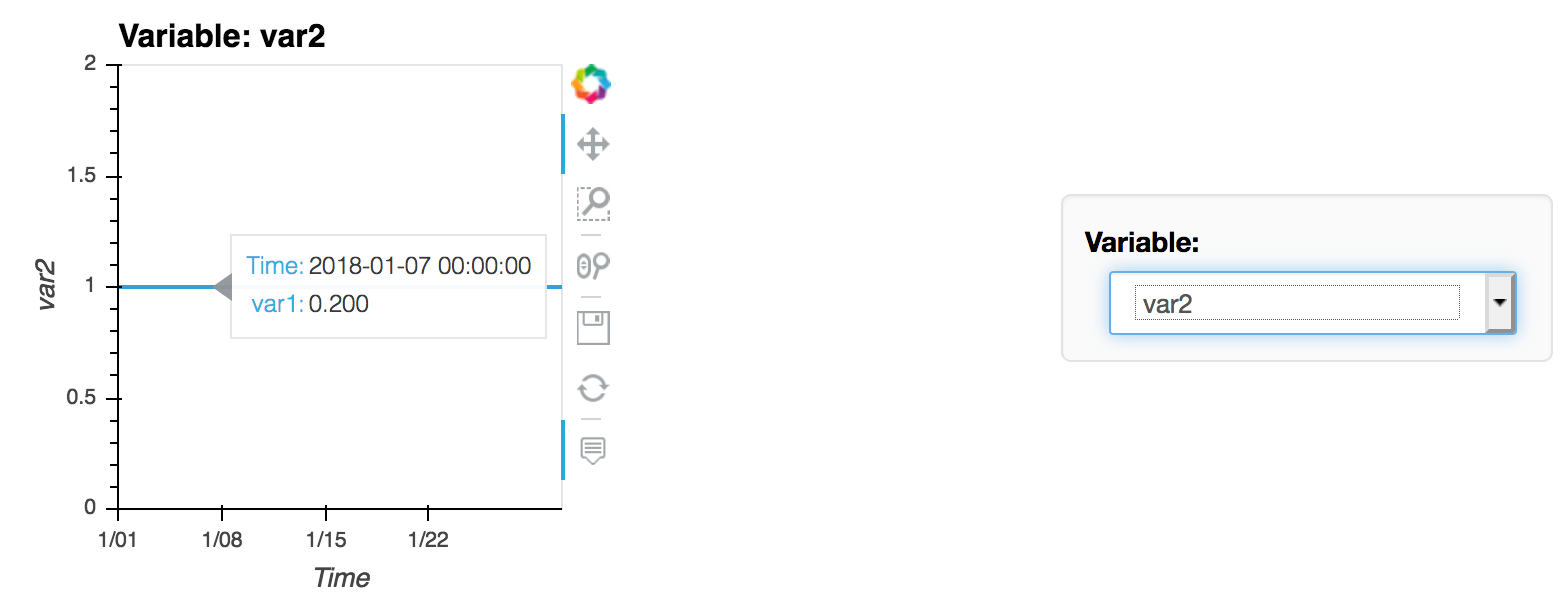{{ message }}

# DynamicMap hover tool does not update with chosen variable#3609

Closed
opened this issue Apr 8, 2019 · 2 comments
Closed

# DynamicMap hover tool does not update with chosen variable#3609

opened this issue Apr 8, 2019 · 2 comments
Labels
Milestone

###dnowacki-usgs commented Apr 8, 2019 • edited Loading

 The following code creates a DynamicMap allowing you to choose from two variables: ``````import holoviews as hv import pandas as pd import numpy as np hv.extension('bokeh') df = pd.DataFrame() df['time'] = pd.date_range('2018-01-01', '2018-01-31') df['var1'] = np.linspace(0, 1, len(df['time'])) df['var2'] = np.ones(df['var1'].shape) def load_symbol(var): return hv.Curve(df, ('time', 'Time'), var) variables = ['var1', 'var2'] dmap = hv.DynamicMap(load_symbol, kdims='Variable').redim.values(Variable=variables) dmap.opts(framewise=True, tools=['hover']) `````` However, the hover tool continues to show the value for var1 even when plotting var2. Correct behavior for var1:Incorrect behavior for var2:The tooltip still shows the var1 value of 0.2, but it should read 1. What's going on here? The text was updated successfully, but these errors were encountered:

###philippjfr commented Apr 8, 2019

 Thank you, this is indeed a bug. Originally HoloViews did not support changing the dimensions of an element, this changed a long time ago but it seems like we never implemented updates to the HoverTool tooltips.

###jorloplaz commented May 30, 2019

 This still doesn't work if one tries to use a custom tooltip instead of the default one. Changing slightly the previous example: ``````import holoviews as hv import pandas as pd import numpy as np hv.extension('bokeh') from bokeh.models import HoverTool df = pd.DataFrame() df['time'] = pd.date_range('2018-01-01', '2018-01-31') df['var1'] = np.linspace(0, 1, len(df['time'])) df['var2'] = np.ones(df['var1'].shape) def load_symbol(var): return hv.Curve(df, [('time', 'Time')], [var]).opts(tools=[HoverTool(tooltips=[('value', f'@{var}')])]) dmap = (hv.DynamicMap(load_symbol, kdims='var').redim.values(var=['var1', 'var2']) .opts(framewise=True)) dmap `````` Makes value not to update. I think this is related to the way the issue was implemented. There's an `'hv_created'` tag in a sort of internal tooltip, which is assumed to always be present. What if the user instead provides another tooltip instance which should be the one to be updated?